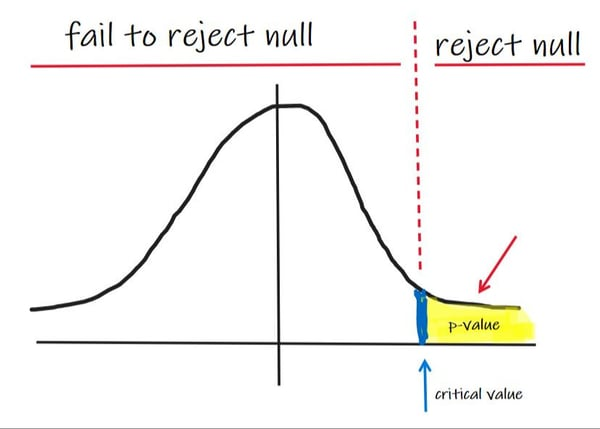Trusted answers to developer questions
Trusted Answers to Developer Questions

Related Tags

data science

# What is p-value in data science?

Hassaan Waqar

Grokking Modern System Design Interview for Engineers & Managers

Ace your System Design Interview and take your career to the next level. Learn to handle the design of applications like Netflix, Quora, Facebook, Uber, and many more in a 45-min interview. Learn the RESHADED framework for architecting web-scale applications by determining requirements, constraints, and assumptions before diving into a step-by-step design process.

p-value is a term widely used in hypothesis testing. By definition, the p-value is the chance under the null hypothesis that the test statistic is equal to the value observed in the data or further in the direction of the alternative. In simpler words, the p-value is the likelihood of finding a particular set of observations if the null hypothesis is true.

To understand the p-value further, we will first need to gain some background knowledge.

## What is hypothesis testing

Hypothesis testing is used in statistics to verify a hypothesis made by researchers or analysts. It is used to test whether findings deduced from some sample data can be generalized to the wider population or not. There are different methods of testing hypotheses. They depend on the nature of the data and the reason for the analysis.

Hypothesis testing begins by stating a null hypothesis and an alternate hypothesis.

The null hypothesis defines the status quo. It states that there is no considerable difference between data obtained before and after an intervention or condition and that any difference occurs merely by chance.

Null hypothesis is represented by $H_{o}$

The alternate hypothesis claims that there is a significant difference between data obtained before and after an intervention or condition and that this difference cannot occur merely by chance.

Alternate hypothesis is represented by $H_{a}$

Both null and alternate hypotheses are mutually exclusive. Only one of them can be true at a time.

Data is then collected and analyzed. Finally, a decision is made regarding the null hypothesis using the p-value, which is calculated using the test statistic. It is either rejected, or researchers fail to reject the null hypothesis with the given data.

Test statistic is a variable that is randomly obtained from sample data. It is used in hypothesis testing.

Another term is necessary to understand the concept of the p-value. That term is significance level

## What is the significance level?

The significance level is the measure of the strength of evidence that must be present in our sample before we can reject the null hypothesis.

Significance level is decided by researchers before the experiment.

The significance level is the probability of rejecting the null hypothesis when it is true. Rejecting the null hypothesis means that we conclude that the results obtained are statistically significant and do not occur merely by chance.

Lower significance levels mean that we require stronger evidence before we reject the null hypothesis.

## Understanding p-value

As discussed earlier, the p-value is the likelihood of finding a particular set of observations if the null hypothesis is true. It is used along with the significance level to decide whether to reject or not reject the null hypothesis.

If the p-value is lesser than the significance level, we reject the null hypothesis. If the p-value is greater than the significance level, we fail to reject the null hypothesis.

The illustration below shows the normal distribution:

Area under the curve is 1.

The critical value divides the curve into two portions: rejecting the null and failing to reject the null.p-value

The critical value is based upon the significance level. The area to the right of the critical value denotes the significance level. If the p-value is lower than the significance value (p-value lies to the right of the critical value), we reject the null hypothesis. If the p-value is greater than the significance level (p-value lies to the left of the critical value), we fail to reject the null hypothesis.

Different methods are used to calculate the p-value depending on the type of data available and the nature of the problem.

RELATED TAGS

data science

CONTRIBUTOR

Hassaan Waqar